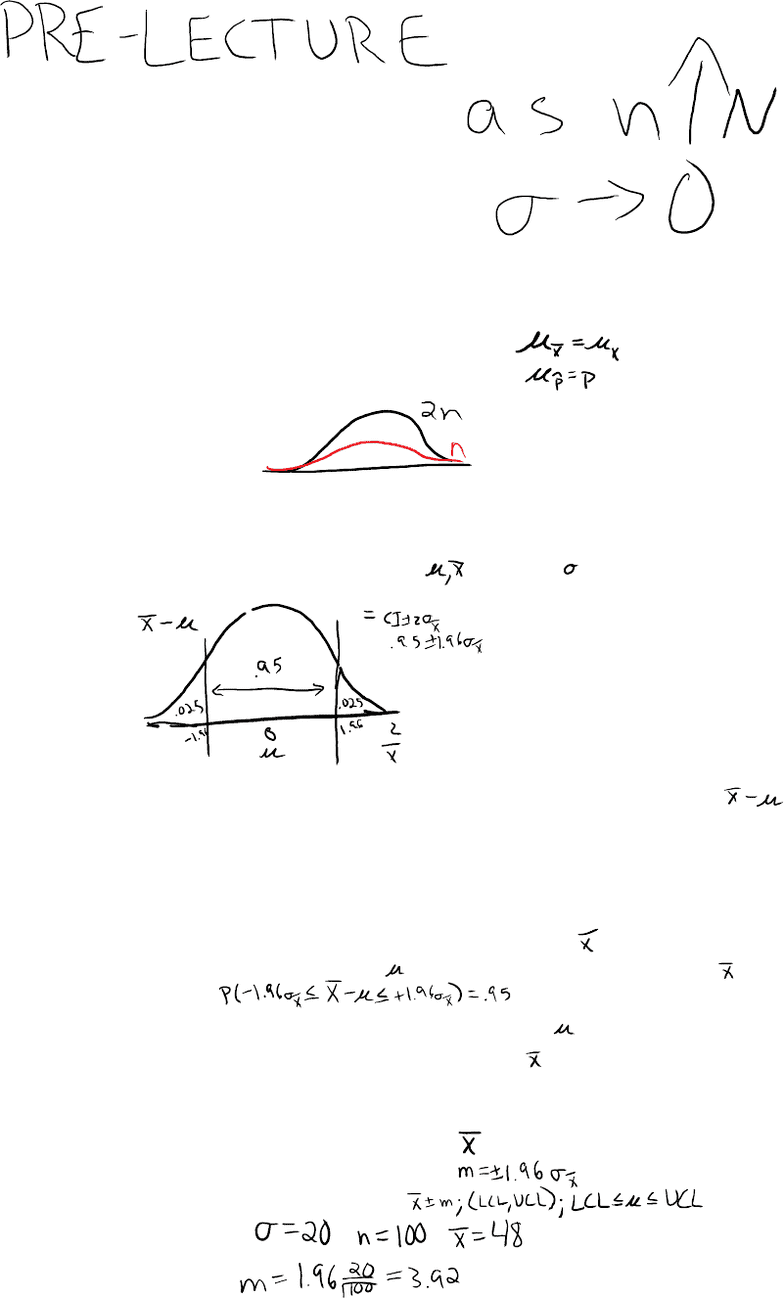# BUSMGT 2320 Lecture 1: 1. 1-Sample Z CI

26 views4 pages

For unlimited access to Class Notes, a Class+ subscription is required.Point estimator: one valuei.
Interval estimator: rangeii.
Estimator = formulaa.
Point estimate: x = 15.2i.
Interval estimate: (11.2, 19.2)ii.
Estimate = valueb.
Estimator vs EstimateI.
The expected value (mean) of the statistic is equal to the population
parameter that you are trying to estimate
i.
Unbiasednessa.
As the sample size increases, the variance of the sampling distribution
decreases
i.
Consistencyb.
A statistic that has lower variability than another is (relatively) more
efficient
i.
Efficiencyc.
Properties of Classical EstimatorsII.
Sampling Error vs. Margin of Errora.
Sampling error is the actual difference between the value of the point
estimate and the value of the parameter it estimates,
i.
Probability1.
Variability 2.
95% of the time the observed value of should not be farther
away from than 1.96 standard deviations of
3.
Margin of Error is the maximum "reasonable" size of the sampling
error
ii.
95% of the time, the actual value of should not be farther
away from the observed value of than 1.96 standard
deviations
4.
Begin with the point estimate:i.
Determine the margin of error:ii.
95% Confidence Interval: iii.
Example:iv.
Calculating the 95% Symmetric CIb.
95% Symmetric Confidence Interval for ~ Normal, knownIII.
1
-
Sample Z CI Module
Monday, January 9, 2017 02:20 PM
Unit 1 Page 1
Unlock document

This preview shows page 1 of the document.
Unlock all 4 pages and 3 million more documents.

Already have an account? Log in

# Get access

Grade+
\$10 USD/m
Billed \$120 USD annually
Homework Help
Class Notes
Textbook Notes
40 Verified Answers
Study Guides
1 Booster Class
Class+
\$8 USD/m
Billed \$96 USD annually
Homework Help
Class Notes
Textbook Notes
30 Verified Answers
Study Guides
1 Booster Class